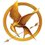# Programming Task 2: Cashier.

Hey guys, here is task number $\boxed{2}$!

As you can see, the name of this is CASHIER.

Plarry the Dinosaur has finally found a job as a cashier! (yay) However, the thing is, he is terrible at mental sum and wants you to write a code to help him in calculating the cost of items.

Input Details:

First line inputs n.

Next n lines input the cost of the ith item.

This line inputs x.

The next x lines are the items the purchaser bought, the number i representing the ith item.

Output Details:

The total cost of all the items.

Sample input:

3

0.25

5.67

10.01

2

3

1

Sample output:

10.26

Explanation for output:

The 1st item costs 0.25 dollars and the 3rd item costs 10.01 dollars. Thus, the total cost is 10.26 dollars.Note by Charlton Teo
6 years, 10 months ago

This discussion board is a place to discuss our Daily Challenges and the math and science related to those challenges. Explanations are more than just a solution — they should explain the steps and thinking strategies that you used to obtain the solution. Comments should further the discussion of math and science.

When posting on Brilliant:

• Use the emojis to react to an explanation, whether you're congratulating a job well done , or just really confused .
• Ask specific questions about the challenge or the steps in somebody's explanation. Well-posed questions can add a lot to the discussion, but posting "I don't understand!" doesn't help anyone.
• Try to contribute something new to the discussion, whether it is an extension, generalization or other idea related to the challenge.
• Stay on topic — we're all here to learn more about math and science, not to hear about your favorite get-rich-quick scheme or current world events.

MarkdownAppears as
*italics* or _italics_ italics
**bold** or __bold__ bold
- bulleted- list
• bulleted
• list
1. numbered2. list
1. numbered
2. list
Note: you must add a full line of space before and after lists for them to show up correctly
paragraph 1paragraph 2

paragraph 1

paragraph 2

[example link](https://brilliant.org)example link
> This is a quote
This is a quote
    # I indented these lines
# 4 spaces, and now they show
# up as a code block.

print "hello world"
# I indented these lines
# 4 spaces, and now they show
# up as a code block.

print "hello world"
MathAppears as
Remember to wrap math in $$ ... $$ or $ ... $ to ensure proper formatting.
2 \times 3 $2 \times 3$
2^{34} $2^{34}$
a_{i-1} $a_{i-1}$
\frac{2}{3} $\frac{2}{3}$
\sqrt{2} $\sqrt{2}$
\sum_{i=1}^3 $\sum_{i=1}^3$
\sin \theta $\sin \theta$
\boxed{123} $\boxed{123}$

Sort by:

Top Newest

My solution in

# C++

  1 2 3 4 5 6 7 8 9 10 11 12 13 14 15 16 17 18 19 20 21 22 23 24 25 26 #include #include using namespace std; int main(){ int items; cin >> items; vector costs; while (items--){ double cost; cin >> cost; costs.push_back(cost); } int bought; cin >> bought; double sum = 0; while (bought--){ int index; cin >> index; sum += costs[index]; } cout << sum; return 0; } 

- 6 years, 10 months ago

Log in to reply

My solution in

# Java

  1 2 3 4 5 6 7 8 9 10 11 12 13 14 15 16 17 18 19 20 21 22 23 24 25 26 27 import java.util.Scanner; public class Cashier { static Scanner in = new Scanner(System.in); public static void main(String args[]) { int items = in.nextInt(); double[] costs = new double[items]; for (int i = 0; i < items; i++) { costs[i] = in.nextDouble(); } int bought = in.nextInt(); double sum = 0; for (int i = 0; i < bought; i++) { int index = in.nextInt(); sum+=costs[index - 1]; } System.out.println(sum); } } 

- 6 years, 10 months ago

Log in to reply

And here is python2 solution below:

  1 2 3 4 5 6 7 8 9 10 11 12 13 14 num = input() # input n price_list = [] # inserting price for ith item for n in xrange(num): price_list.append(raw_input()) items = input() # no of item bought cost = 0 # summing up prices for item in xrange(items): cost += float(price_list[input()-1]) print cost 

- 6 years, 10 months ago

Log in to reply

sorry ur the only one who tried this daniel XD anyway my solution:

  1 2 3 4 5 6 7 8 9 10 11 12 13 14 15 16 17 18 19 20 21 #include using namespace std; int main() { int x,n; double p; double b = 0; cin>>x; for(int i = 0; i < x; i++){ cin>>p[i]; } cin>>n; for(int i = 0; i < n; i++){ int a; cin>>a; b+=p[a-1]; } cout<

- 6 years, 10 months ago

Log in to reply

maybe no one saw this post

- 6 years, 10 months ago

Log in to reply

×

Problem Loading...

Note Loading...

Set Loading...